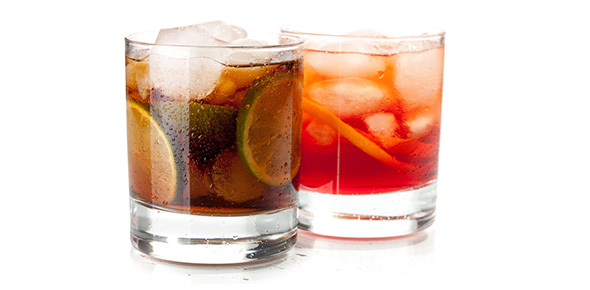# Classifying The Compound: Alcohol

10 Questions | Total Attempts: 82SettingsStudents will take this brief quiz to evaluate their knowledge and familiarity of the compound alcohol based upon their understanding of the provided tutorial on alcohols. From the tutorial they should be able to successfully complete questions based on the general formula, basic properties, nomenclature, and classification of alcohols.

• 1.
What is the name of the functional group including a cyclohexane with a hydroxyl group (-OH) attached?
• A.

Dehydrogenase

• B.

Cyclic alcohol

• C.

Phenol

• D.

Hydroxyhexane

• E.

Amino

• 2.
The boiling point of alcohols is higher than other functional groups (i.e. hydrocarbons and ethers) due to what type of bonding?
• A.

Hydrogen

• B.

Ionic

• C.

Double bonds

• D.

Covalent

• E.

B & D

• 3.
Name the following compound:  CH3CH2OH
• A.

N- propyl alcohol

• B.

Methanol

• C.

Glycol

• D.

Ethanol

• E.

Tert- butyl alcohol

• 4.
How many "R" groups does a tertiary alcohol contain?
• A.

0

• B.

1

• C.

2

• D.

3

• E.

4

• 5.
How does extending the alkyl chain affect pKa in alcohols?
• A.

Lowers pKa

• B.

Raises pKa

• C.

Extending the alkyl chain does not affect pKa

• D.

Extending the alkyl chain stabilizes pKa

• E.

None of the above

• 6.
Reacting an alochol with a strong base results in?
• A.

A strong acid

• B.

Breaking hydrogen bonds

• C.

A hydrophobic alcohol

• D.

A dehydration

• E.

An alkoxide salt

• 7.
Water solubility in alcohols decreases as the carbon chain increases in length to how many carbons?
• A.

4-5

• B.

6-7

• C.

8-10

• D.

12-15

• E.

20+

• 8.
A hydrogen bond is an interaction between what two things?
• A.

Strongly acidic hydrogen; a weak base

• B.

• C.

Weakly acidic hydrogen; lone pair of electrons

• D.

Two hydroxyl groups

• E.

Strong acid; strong base

• 9.
What makes the alcohol molecule polar?
• A.

Hydrogen bonding

• B.

Hydroxyl group

• C.

Van der Waal forces

• D.

Intermolecular forces

• E.

Alkyl chain

• 10.
Each hydrogen bond has an energy of?
• A.

-20 Kcal/mol

• B.

-10 Kcal/mol

• C.

1-2 Kcal/mol

• D.

4-5 Kcal/mol

• E.

None of the above

Related TopicsBack to top